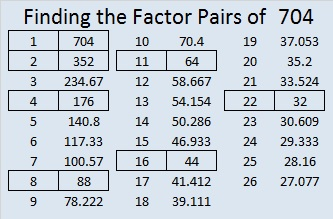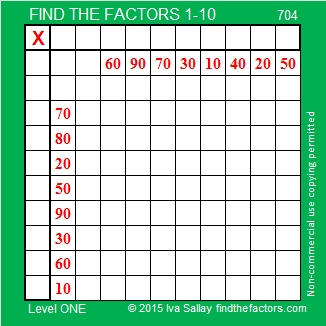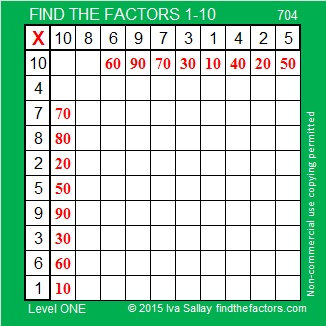# 704 and Level 1

• 704 is a composite number.
• Prime factorization: 704 = 2 ⋅ 2 ⋅ 2 ⋅ 2 ⋅ 2 ⋅ 2 ⋅ 11, which can be written 704 = (2^6) ⋅ 11
• The exponents in the prime factorization are 6, and 1. Adding one to each and multiplying we get (6 + 1)(1 + 1) = 7 ⋅ 2 = 14. Therefore 704 has exactly 14 factors.
• Factors of 704: 1, 2, 4, 8, 11, 16, 22, 32, 44, 64, 88, 176, 352, 704
• Factor pairs: 704 = 1 x 704, 2 x 352, 4 x 176, 8 x 88, 11 x 64, 16 x 44, or 22 x 32
• Taking the factor pair with the largest square number factor, we get √704 = (√64)(√11) = 8√11 ≈ 26.532998.This Level 1 puzzle is not difficult at all:Print the puzzles or type the solution on this excel file: 10 Factors 2015-12-07

———————————————————————————

Here are a few more thoughts about the number 704:

7 – 0 + 4 = 11 so 704 is divisible by 11.

Here’s a fun multiplication fact: 8 x 88 = 704

Also 704 is a palindrome in two different bases:

• 1I1 in BASE 19; note 19² = 361, I is equivalent to 18 base 10, and 1(361) + 18(19) + 1(1) = 704
• MM in BASE 31; note M is equivalent to 22 base 10, and 22(31) = 22(1) = 704

———————————————————————————This site uses Akismet to reduce spam. Learn how your comment data is processed.